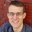Question-and-Answer Resource for the Building Energy Modeling Community
Get started with the Help page

# remove curve via openstudio measureI have created a custom VRF systems & terminals measure for Daikin units. I have curves only where absolutely necessary, and where possible, I am referring to common curves. For example, two different AirConditioner:VariableRefrigerantFlow objects can have the same CoolCapFT curve, so there should not be a need to create a CoolCapFT 1 curve for one and CoolCapFT curve 2 for the other - it unnecessarily clutters the .osm file.

Nevertheless, it seems that upon creation of a, for example, AirConditioner:VariableRefrigerantFlow, OpenStudio automatically creates all kinds of curves, even though later on I reassign a consistent set of curves and these curves then become obsolete.

So I thought I'd just develop a curves clean-up measures. I noticed that these annoyance curves all have similar names and then an incremental counter appended to them, so I just look for curves that contain that common name sub-string and remove them. The curves are definitely recognized, but for some reason, not removed from the .osm file when I run the measure, though.

For example, in measure.rb, I do the following:

curve_names = []
curve_names << "VRFCoolCapFT"
curve_names << "VRFCoolCapFTBoundary"
curve_names << "VRFCoolCapFTHi"
curve_names << "VRFCoolEIRFT"
curve_names << "VRFCoolEIRFTBoundary"
curve_names << "VRFCoolEIRFTHi"
curve_names << "CoolingEIRLowPLR"
curve_names << "CoolingEIRHiPLR"
curve_names << "CoolingCombRatio"
curve_names << "CoolingLengthCorrectionFactor"

curve_names << "VRFHeatCapFT"
curve_names << "VRFHeatCapFTBoundary"
curve_names << "VRFHeatCapFTHi"
curve_names << "VRFHeatEIRFT"
curve_names << "VRFHeatEIRFTBoundary"
curve_names << "VRFHeatEIRFTHi"
curve_names << "HeatingEIRLowPLR"
curve_names << "HeatingEIRHiPLR"
curve_names << "HeatingCombRatio"
curve_names << "HeatingLengthCorrectionFactor"

curve_names << "VRFTUCoolCapFT"
curve_names << "VRFACCoolCapFFF"
curve_names << "VRFTUHeatCAPFT"
curve_names << "VRFACHeatCapFFF"

curve_names << "Fan On Off Power Curve"
curve_names << "Fan On Off Efficiency Curve"

deletecurves(runner, model, curve_names)


And that last function is defined as follows:

def deletecurves(runner, model, curve_names)
curve_names.each do |curve_name|
end
end
end

linear_curves = model.getObjectsByType(OpenStudio::Model::CurveLinear::iddObjectType)
linear_curves.each do |linear_curve|
linear_curve_name = linear_curve.name.get
curve_names.each do |curve_name|
if linear_curve_name.include? curve_name then
puts "Will delete Linear: #{linear_curve.name}\n"
linear_curve.remove
end
end
end

curve_names.each do |curve_name|
end
end
end

cubic_curves = model.getObjectsByType(OpenStudio::Model::CurveCubic::iddObjectType)
cubic_curves.each do |cubic_curve|
cubic_curve_name = cubic_curve.name.get
curve_names.each do |curve_name|
if cubic_curve_name.include? curve_name then
puts "Will delete Cubic: #{cubic_curve.name}\n"
cubic_curve.remove
end
end
end

exponent_curves = model.getObjectsByType(OpenStudio::Model::CurveExponent::iddObjectType)
exponent_curves.each do |exponent_curve|
exponent_curve_name = exponent_curve.name.get
curve_names.each do |curve_name|
if exponent_curve_name.include? curve_name then
puts "Will delete Exponent: #{exponent_curve.name}\n"
exponent_curve.remove
end
end
end


I'd appreciate any hints for resolving this issue.

edit retag close merge delete

Sort by » oldest newest most votedTake a look at the Remove Orphan Objects and Unused Resources measure on BCL for an example.

OpenStudio::Model::Curve objects are a child of OpenStudio::Model::ResourceObject [link to documentation], which has a directUseCount method.

So your code could look as simple as:

  model.getCurves.sort.each do |curve|
if curve.directUseCount == 0
model.removeObject(curve.handle)
end
end


I've added curves to the Remove Orphan Objects and Unused Resources measure. The updated version should be on BCL in the next week or so, or you can grab it from GitHub here.

EDIT: use model.removeObject instead of curve.remove because of this issue. Thanks to @Eric Ringold for the workaround.

more

Thank you, mdahlhausen. I copied your method, but unfortunately, it still does not delete those curves, even though it clearly finds all 663 (!) of them. Just for good measure, when looking through the .osm file and searching for references to the handles of these curves, there are none, so they really are orphans, and there should not even be knowledge there that these belonged to a VRF system or VRF terminal, in case such association should prevent them from being deleted. This should be easy, but is not. I am at a loss. Is there another function beside .remove?

@mdahlhausen sure seems like a bug with curves that prevents them from being removed, even if unused in the model. Try adding a curve to an empty model in irb. The curve will respond true to curve.parent.empty? and 0 to curve.directUseCount, but will not be removed with curve.remove. In contrast, other ResourceObjects (I tried Construction and DefaultConstructionSet) will be removed.

Thanks @Eric Ringold. I'll take a look at src to see what is happening. [update:] curve.remove should remove the curve but doesn't. It's a bug in OpenStudio. Issue filed.

Yeah there's a lot going on here (called from WorkspaceObject_Impl::remove()) that I couldn't track, at least that would cause any meaningful difference between ResourceObject types.

@Matt Koch: see here for a method that will work. Added to the issue as a workaround to .remove.